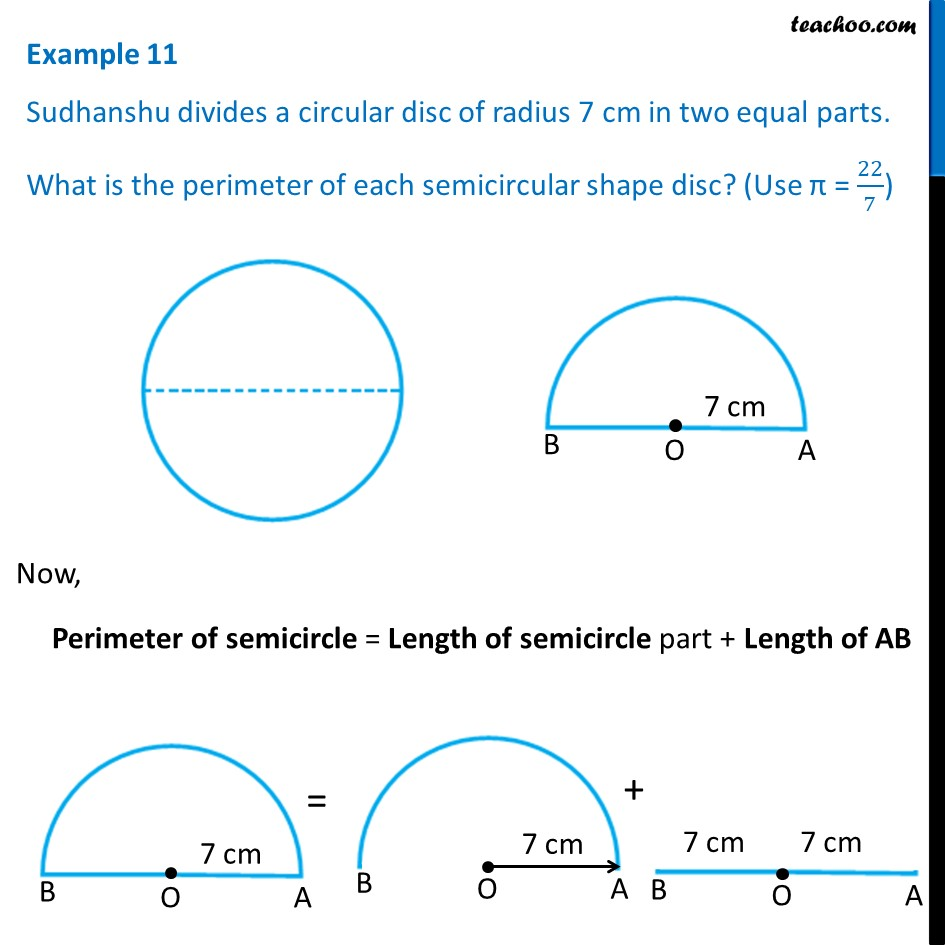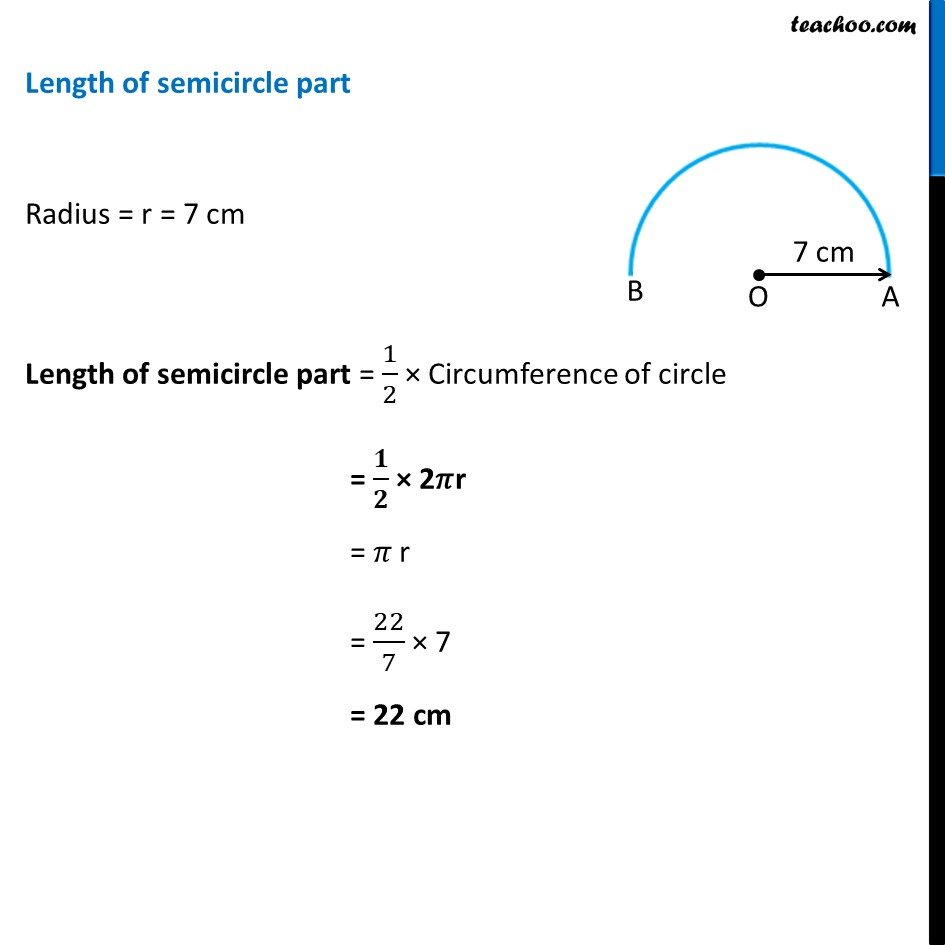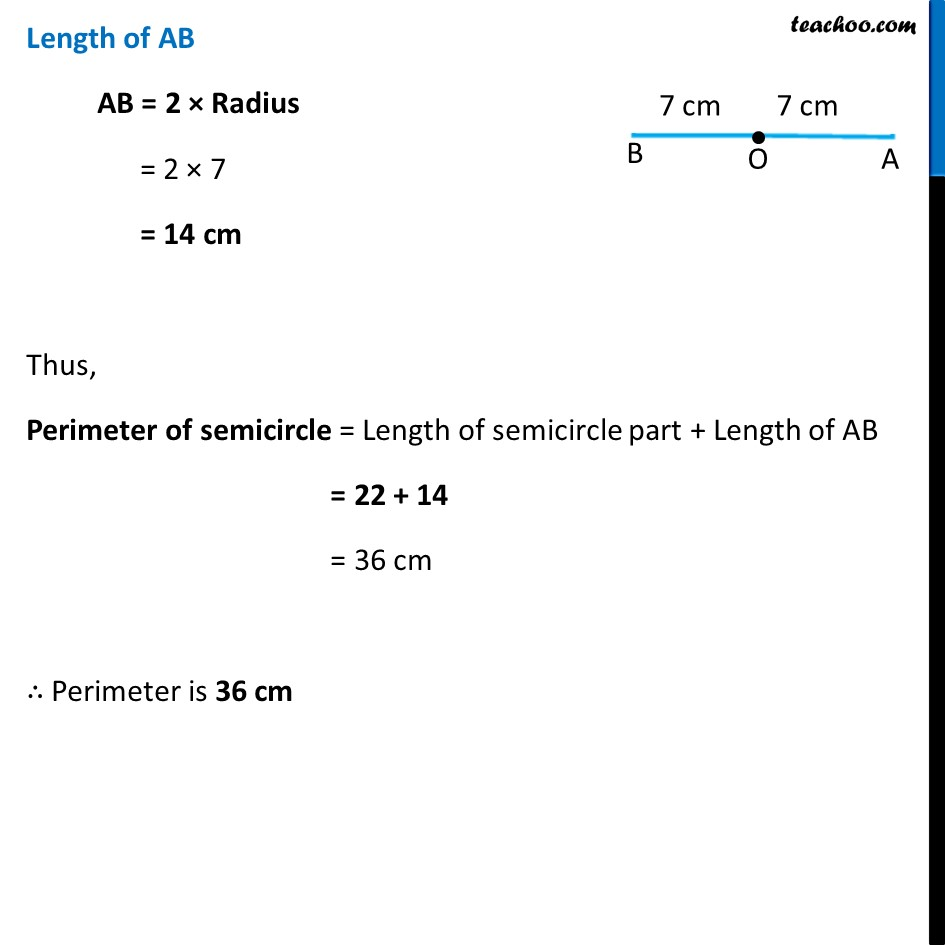Examples

Chapter 9 Class 7 Perimeter and Area
Serial order wiseLearn in your speed, with individual attention - Teachoo Maths 1-on-1 Class

### Transcript

Example 11 Sudhanshu divides a circular disc of radius 7 cm in two equal parts. What is the perimeter of each semicircular shape disc? (Use π = 22/7)Now, Perimeter of semicircle = Length of semicircle part + Length of AB Length of semicircle part Radius = r = 7 cm Length of semicircle part = 1/2 × Circumference of circle = 𝟏/𝟐 × 2𝜋r = 𝜋 r = 22/7 × 7 = 22 cm Length of AB AB = 2 × Radius = 2 × 7 = 14 cm Thus, Perimeter of semicircle = Length of semicircle part + Length of AB = 22 + 14 = 36 cm ∴ Perimeter is 36 cm